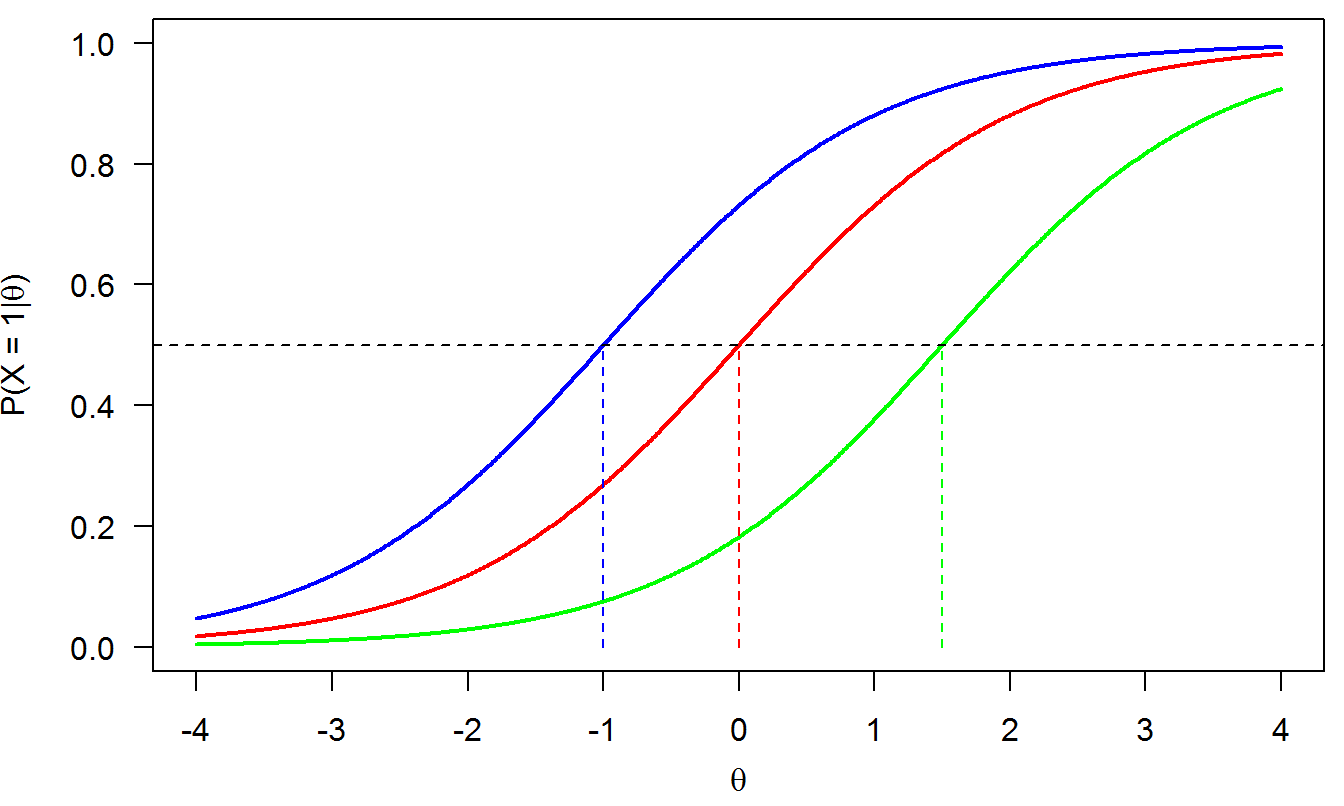# 2 The Rasch model (1PLM)

The item response function (IRF) for item $$i$$ is given by

$\begin{equation} P(X_i=1|\theta) = \frac{\exp(\theta - \delta_i)}{1 + \exp(\theta-\delta_i)}, \tag{2.1} \end{equation}$

where

• $$\theta$$ is the person’s latent ability parameter;

• $$\delta_i$$ is the item’s difficulty or popularity.

This IRF is known as the one-parameter logistic model because it involves only one item parameter (difficulty $$\delta$$) and its functional form is based on the logistic function.

Figure 2.1 shows three examples of IRFs under the Rasch model for three difficulty levels: $$\delta = -1$$ (blue), $$\delta = 0$$ (red), and $$\delta = 1.5$$ (green).Figure 2.1: 1PLM (Rasch model)

Properties of IRFs from the Rasch model:

• The probabilities increase with the latent trait $$\theta$$ for each item. So, the higher the latent trait, the higher the probability of answering the item correctly.

• The curves are ‘parallel’, that is, they do not intersect.

• Items differ only in difficulty: Easier items are on the left (low difficuly $$\delta$$, like the blue item) and difficult items are on the right (high difficulty $$\delta$$, like to green item).

• Parameter $$\delta$$ is the value of latent trait for which the probability of answering the item correctly is 50%: $$P(X = 1|\theta=\delta) = .50$$.